# Knowledge Base

## Solution 34591: Using the Numeric Solver on the TI-84 Plus CE and TI-84 Plus C Silver Edition.

### How do I solve equations on the TI-84 Plus CE and TI-84 Plus C Silver Edition?

The example below will demonstrate how to use the Numeric Solver feature.

Example: Solve for x if 2x+6=10

The TI-84 Plus CE and TI-84 Plus C Silver Edition can display either Mathprint or Classic modes to solve equations. Mathprint is the default mode, to verify or change modes, press [MODE], highlight MATHPRINT or CLASSIC and press [ENTER].

To solve 2x+6=10 in Mathprint mode, follow the steps below:

1) Press [MATH][ALPHA][B] OR press [MATH] and scroll down to B:Solver...and press [ENTER].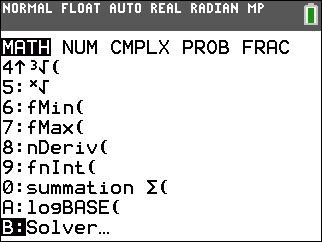2) Input 2x+6 in the E1 (Expression1) box and press [ENTER].
3) Input 10 in the E2 (Expression2) box and press [ENTER].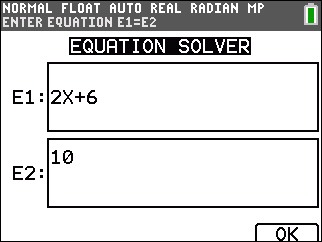4) Highlight x and press [ALPHA][SOLVE] to solve the equation for x.

The display will show x=2.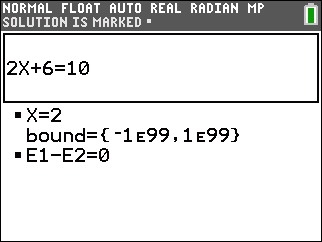To solve 2x+6=10 in Classic mode, follow the steps below:

1) Press [MATH][ALPHA][B] OR press [MATH] and scroll down to B:Solver...and press [ENTER].

Please Note: In Classic mode, the numeric "Solver" is used to solve equations in the form 0=; therefore, any equation that is entered would need to equal zero.

2) Input 2x+6-10 after eqn:0=, and press [ENTER].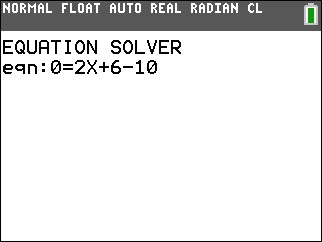3) Highlight x and press [ALPHA][SOLVE] to solve the equation for x.

The display will show x=2.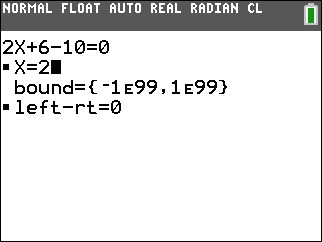Terminology and Miscellaneous Notes:

• bound={lower,upper}. lower and upper are the bounds between which the TI-84 Plus CE and TI-84 Plus C Silver Edition searches for a solution. This is optional, but it may help find the solution more quickly. The default is bound= {-1E99, 1E99}
• The solution is displayed next to the variable for which you solved. A solid square in the first column marks the variable for which you solved and indicates that the equation is balanced. An ellipsis shows that the value continues beyond the screen.
• When a number continues beyond the screen, be sure to press the right arrow (-->) to scroll to the end of the number to see whether it ends with a negative or positive exponent. A very small number may appear to be a large number until you scroll right to see the exponent
• The values of the variables are updated in memory.
• left-rt=diff is displayed in the last line of the editor. diff is the difference between the left and right sides of the equation when evaluated at the calculated solution. A solid square in the first column next to left-rt indicates that the equation has been evaluated at the new value of the variable for which you solved

Please see the TI-84 Plus CE and TI-84 Plus C Silver Edition guidebooks for additional information.